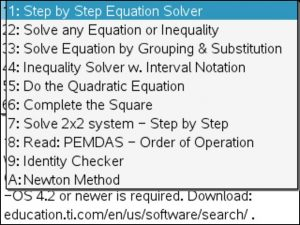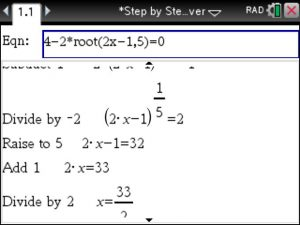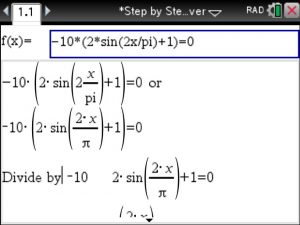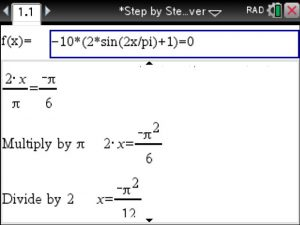## ▷Solve Equation with Radicals ( Roots )- Step by Step – using the TiNSpire CX CAS

Using the 1. menu option in the Step by Step Equation Solver app at www.tinspireapps.com :Just enter the radical using the root() command.

To enter the 5th root of 2x-1 , type in root(2x-1,5) as shown below:All steps are automatically shown until thePosted on Categories equation, radical, steps

## VIDEO: Solve 2×2 System of Equations in Algebra using the TiNspire – Step by Step

Here is a brief video showing how to solve any 2×2 system of equations in Algebra using the TiNspire calculator – best of all : each step of the elimination method is shown.  This handy Algebra Solver is available in Algebra Made Easy at www.TiNspireApps.com  .

Posted on Categories algebra, equation, solver

## Solve any Equation – Step by Step – using the TiNspire

Using the Step by Step Equation Solver APP from www.TiNspireApps.com  for the TiNspire CX CAS you select
the first option: 1: Step by Step Equation SolverEnter any equation and view the steps until final answer is derived.Since we are solving a trigonometric (thus periodic) equation in above example
we obtain infinity many solutions whose setup is displayed in the
last line. n11 represents a constant, think of it as constant K as typically used in text books.
If you set n11 equal to 0 for the first expression for the solution x you recognize the solution
derived step by step.

Posted on Categories equation, solver, tinspirecx